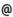## Babes-Bolyai University of Cluj-Napoca Faculty of Mathematics and Computer Science Study Cycle: Graduate SUBJECT

 Code Subject MMA0015 Discrete Optimisation
 Section Semester Hours: C+S+L Category Type Applied Mathematics 6 2+1+0 speciality optional
 Teaching Staff in Charge
 Prof. LUPSA Liana, Ph.D.,  llupsamath.ubbcluj.ro
 Aims The knowing of some solving methods of a discrete optimizatiom problems, as well as some particular classes of discrete optimization problems. The identification of some practical applications.
 Content 1. Mathematical models. The formulation of the discrete optimization problem. 2. The use of d-base in the study of optimization problems having integer variables. Discrete optimization problems: the study of an optimal solution; reoptimizations, parametrizations. Nonlinear discrete optimization problems. 3. Section type methods for solving discrete linear optimization problems; Gomory@s discrete and continuous algorithm; Young@s algorithm. 4. Branch and bound methods for solving discrete optimization problems. The use of dynamical programming in solving discrete optimization problems. 6. The study of some special classes of discrete optimization problems.
 References 1) Blaga L., Lupsa L., Cercetare operationala. Cluj-Napoca: Ed. Argonaut, 2006. 2) Kaufmann A., Henry-Labordere A., Metode si modele ale cercetarii operationale (Programare in numere intregi). vol. III. Bucuresti: Ed. Stiintifica si Enciclopedica, 1975. 3) Lupsa L., Numerical Optimization Methods. Special issue in discrete optimization. Cluj-Napoca: Ed. Risoprint, 2005. 4) Mokus J., A set of Examples of Global and Discrete Optimization. Application of Bayuesian Heuristic Approach. Dordrecht - Boston - London: Kluwer Academic Publishers, 2000. 5) Taha H.A., Integer programming. Theory, Applications and Computations. New York - San Francisco - London: Academic Press, 1975.
 Assessment Exam and project
 Links: Syllabus for all subjects Romanian version for this subject Rtf format for this subject